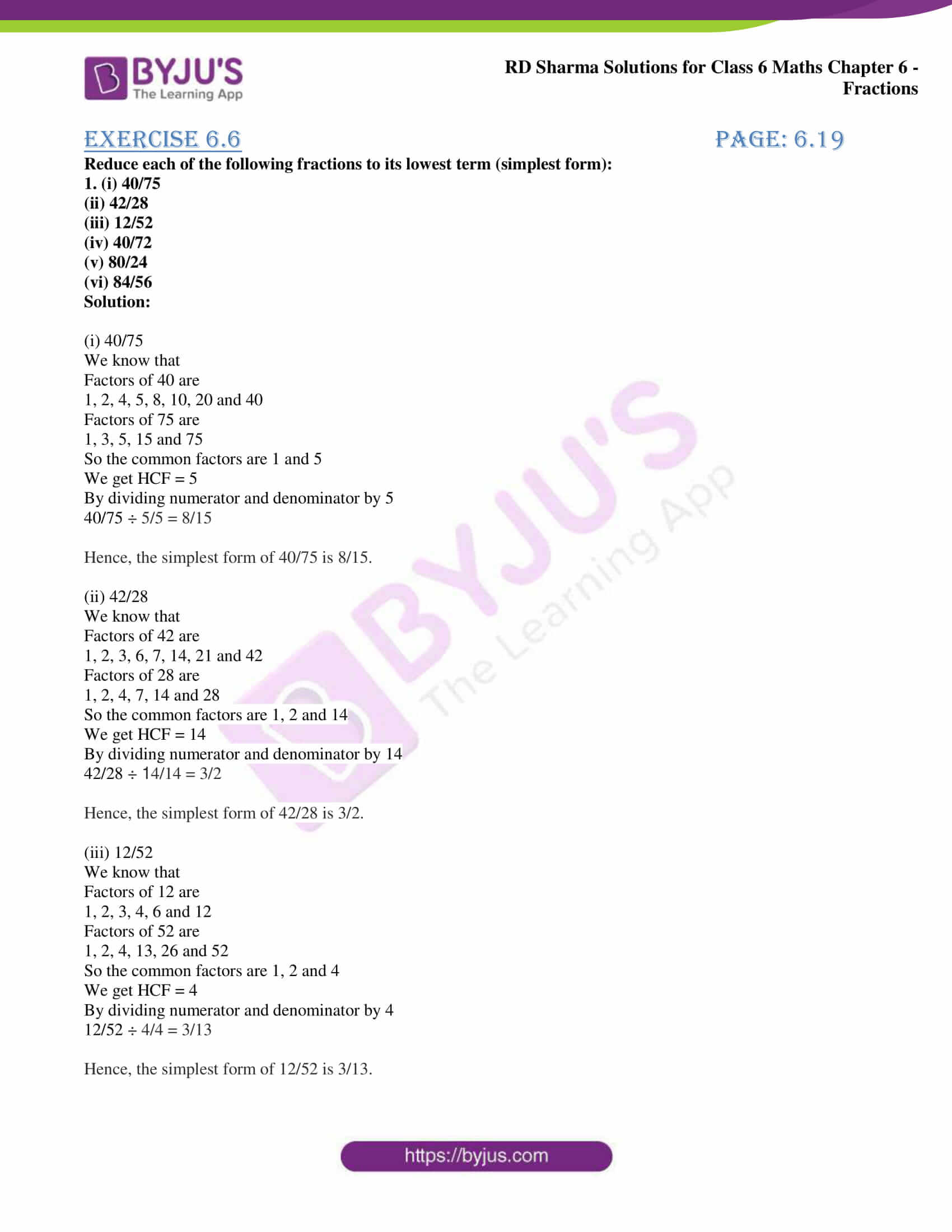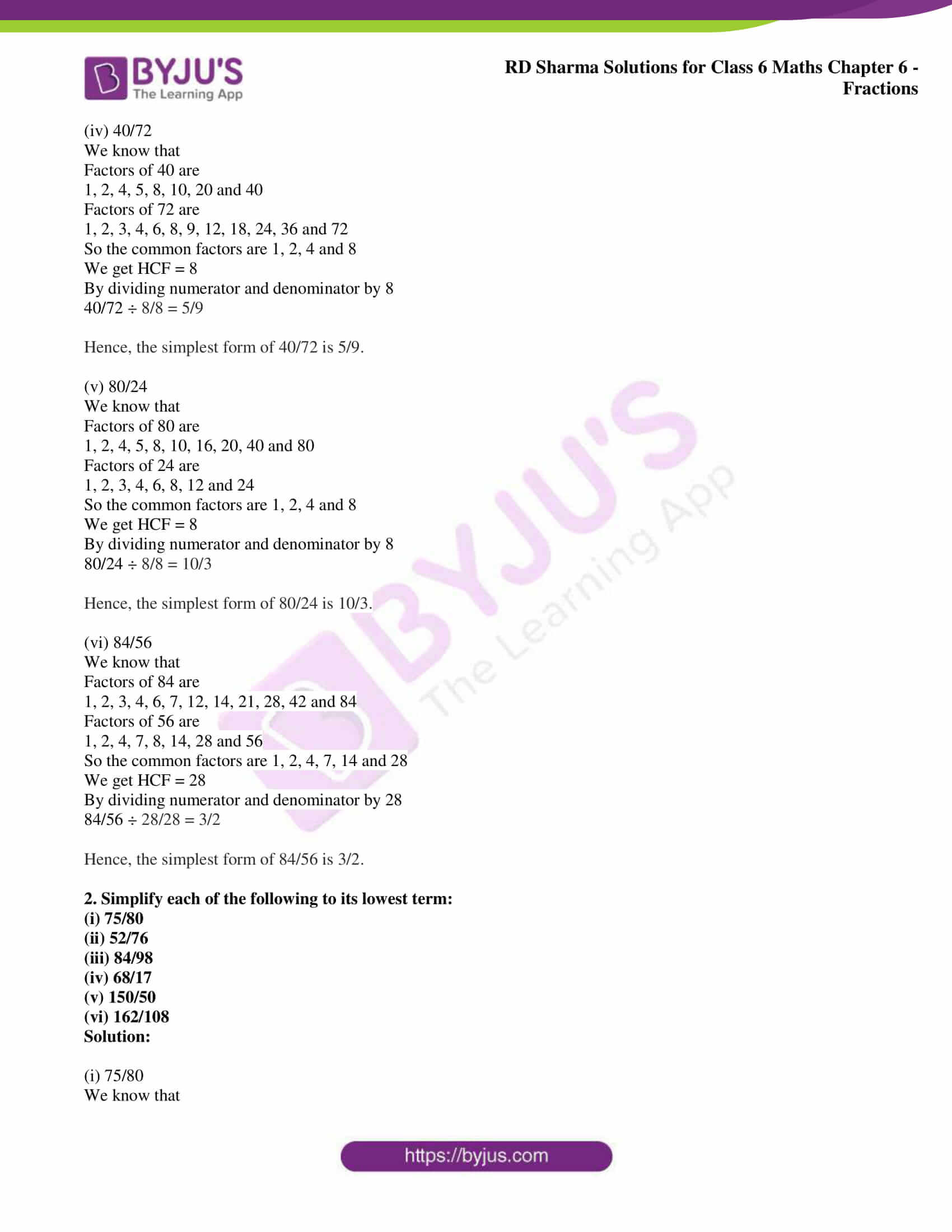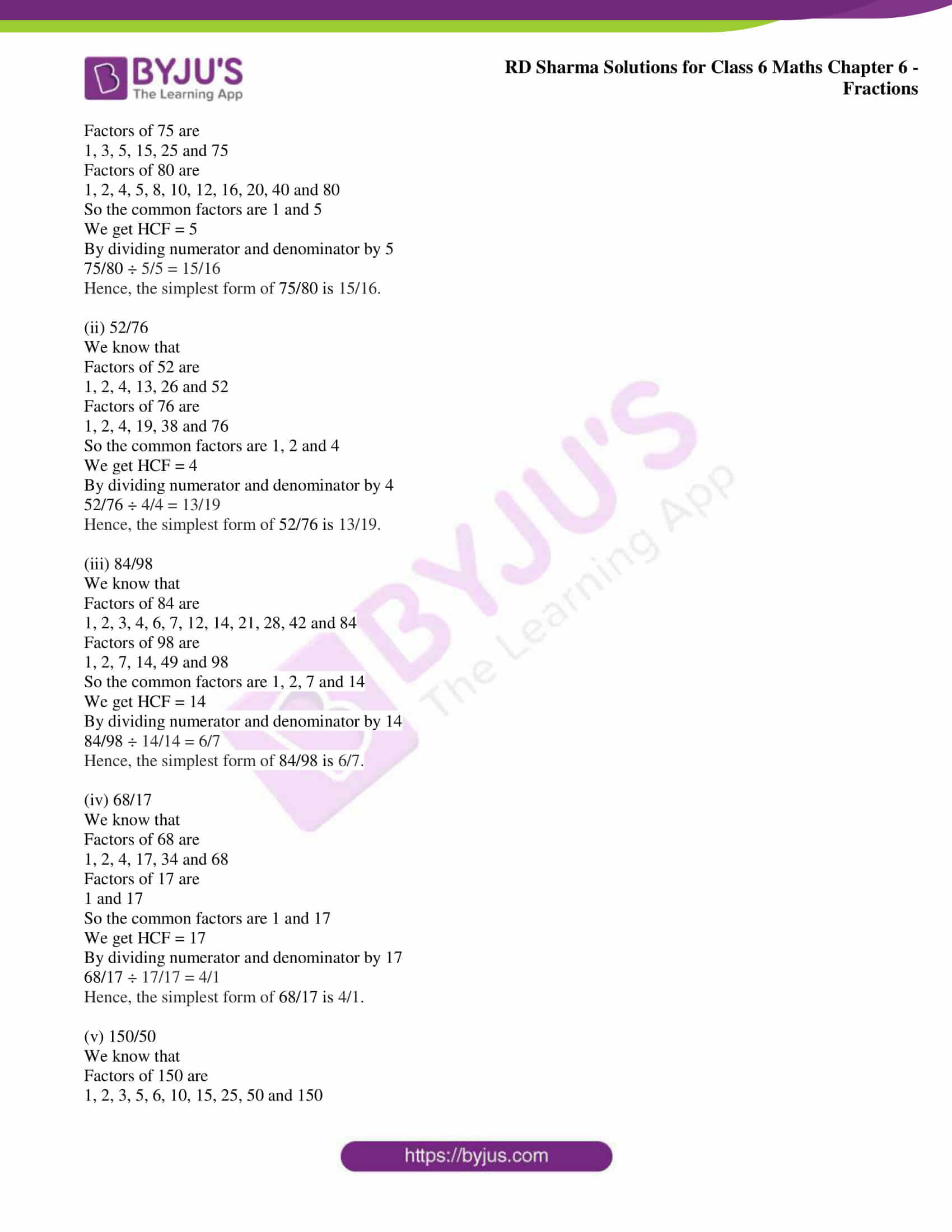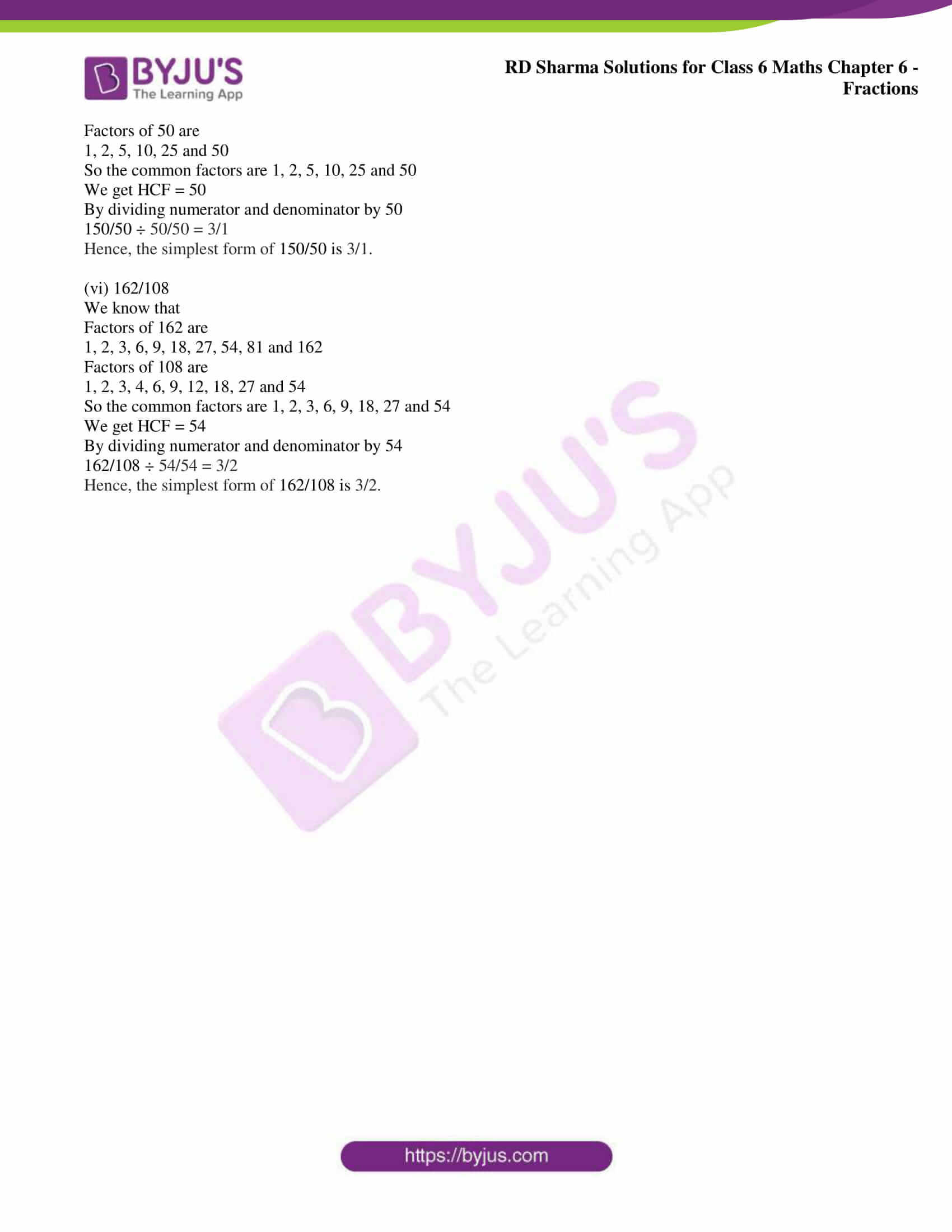# RD Sharma Solutions for Class 6 Maths Chapter 6: Fractions Exercise 6.6

The students must build up better conceptual knowledge from Class 6 as it is significant to their better performance in higher classes. RD Sharma Solutions Class 6 exercise wise problems are prepared by the faculty at BYJU’S which is a major reference guide to ace the exam. This exercise consists of steps of reducing the given fraction to their lowest terms. Students can get their doubts cleared and self analyse the areas which require more time according to the CBSE marks weightage. RD Sharma Solutions for Class 6 Maths Chapter 6 Fractions Exercise 6.6 PDF prepared by the subject matter experts are provided here.

## RD Sharma Solutions for Class 6 Maths Chapter 6: Fractions Exercise 6.6 Download PDF### Access answers to Maths RD Sharma Solutions for Class 6 Chapter 6: Fractions Exercise 6.6

Reduce each of the following fractions to its lowest term (simplest form):

1. (i) 40/75

(ii) 42/28

(iii) 12/52

(iv) 40/72

(v) 80/24

(vi) 84/56

Solution:

(i) 40/75

We know that

Factors of 40 are

1, 2, 4, 5, 8, 10, 20 and 40

Factors of 75 are

1, 3, 5, 15 and 75

So the common factors are 1 and 5

We get HCF = 5

By dividing numerator and denominator by 5

40/75 ÷ 5/5 = 8/15

Hence, the simplest form of 40/75 is 8/15.

(ii) 42/28

We know that

Factors of 42 are

1, 2, 3, 6, 7, 14, 21 and 42

Factors of 28 are

1, 2, 4, 7, 14 and 28

So the common factors are 1, 2 and 14

We get HCF = 14

By dividing numerator and denominator by 14

42/28 ÷ 14/14 = 3/2

Hence, the simplest form of 42/28 is 3/2.

(iii) 12/52

We know that

Factors of 12 are

1, 2, 3, 4, 6 and 12

Factors of 52 are

1, 2, 4, 13, 26 and 52

So the common factors are 1, 2 and 4

We get HCF = 4

By dividing numerator and denominator by 4

12/52 ÷ 4/4 = 3/13

Hence, the simplest form of 12/52 is 3/13.

(iv) 40/72

We know that

Factors of 40 are

1, 2, 4, 5, 8, 10, 20 and 40

Factors of 72 are

1, 2, 3, 4, 6, 8, 9, 12, 18, 24, 36 and 72

So the common factors are 1, 2, 4 and 8

We get HCF = 8

By dividing numerator and denominator by 8

40/72 ÷ 8/8 = 5/9

Hence, the simplest form of 40/72 is 5/9.

(v) 80/24

We know that

Factors of 80 are

1, 2, 4, 5, 8, 10, 16, 20, 40 and 80

Factors of 24 are

1, 2, 3, 4, 6, 8, 12 and 24

So the common factors are 1, 2, 4 and 8

We get HCF = 8

By dividing numerator and denominator by 8

80/24 ÷ 8/8 = 10/3

Hence, the simplest form of 80/24 is 10/3.

(vi) 84/56

We know that

Factors of 84 are

1, 2, 3, 4, 6, 7, 12, 14, 21, 28, 42 and 84

Factors of 56 are

1, 2, 4, 7, 8, 14, 28 and 56

So the common factors are 1, 2, 4, 7, 14 and 28

We get HCF = 28

By dividing numerator and denominator by 28

84/56 ÷ 28/28 = 3/2

Hence, the simplest form of 84/56 is 3/2.

2. Simplify each of the following to its lowest term:

(i) 75/80

(ii) 52/76

(iii) 84/98

(iv) 68/17

(v) 150/50

(vi) 162/108

Solution:

(i) 75/80

We know that

Factors of 75 are

1, 3, 5, 15, 25 and 75

Factors of 80 are

1, 2, 4, 5, 8, 10, 12, 16, 20, 40 and 80

So the common factors are 1 and 5

We get HCF = 5

By dividing numerator and denominator by 5

75/80 ÷ 5/5 = 15/16

Hence, the simplest form of 75/80 is 15/16.

(ii) 52/76

We know that

Factors of 52 are

1, 2, 4, 13, 26 and 52

Factors of 76 are

1, 2, 4, 19, 38 and 76

So the common factors are 1, 2 and 4

We get HCF = 4

By dividing numerator and denominator by 4

52/76 ÷ 4/4 = 13/19

Hence, the simplest form of 52/76 is 13/19.

(iii) 84/98

We know that

Factors of 84 are

1, 2, 3, 4, 6, 7, 12, 14, 21, 28, 42 and 84

Factors of 98 are

1, 2, 7, 14, 49 and 98

So the common factors are 1, 2, 7 and 14

We get HCF = 14

By dividing numerator and denominator by 14

84/98 ÷ 14/14 = 6/7

Hence, the simplest form of 84/98 is 6/7.

(iv) 68/17

We know that

Factors of 68 are

1, 2, 4, 17, 34 and 68

Factors of 17 are

1 and 17

So the common factors are 1 and 17

We get HCF = 17

By dividing numerator and denominator by 17

68/17 ÷ 17/17 = 4/1

Hence, the simplest form of 68/17 is 4/1.

(v) 150/50

We know that

Factors of 150 are

1, 2, 3, 5, 6, 10, 15, 25, 50 and 150

Factors of 50 are

1, 2, 5, 10, 25 and 50

So the common factors are 1, 2, 5, 10, 25 and 50

We get HCF = 50

By dividing numerator and denominator by 50

150/50 ÷ 50/50 = 3/1

Hence, the simplest form of 150/50 is 3/1.

(vi) 162/108

We know that

Factors of 162 are

1, 2, 3, 6, 9, 18, 27, 54, 81 and 162

Factors of 108 are

1, 2, 3, 4, 6, 9, 12, 18, 27 and 54

So the common factors are 1, 2, 3, 6, 9, 18, 27 and 54

We get HCF = 54

By dividing numerator and denominator by 54

162/108 ÷ 54/54 = 3/2

Hence, the simplest form of 162/108 is 3/2.